Latest Banking jobs   »

# Reasoning Quiz For RBI Grade B Phase 1 2023-11th May

Directions (1-2): Following questions are based on the information given below.
‘P × Q’ means ‘P is father of Q’
‘P – Q’ means ‘P is sister of Q’
‘P + Q’ means ‘P is mother of Q’
‘P ÷ Q’ means ‘P is the brother of Q’

Q1. In the expression ‘A × R –G + D ÷ N’, how is D related to A?
(a) Granddaughter
(b) Son
(c) Grandson
(d) Brother
(e) None of these

Q2. Which of the following represents ‘Q is son of U’?
(a) Q ÷ I – G × U
(b) Q + I – G × U
(c) Q ÷ M – N × U
(d) Q ÷ J – N + U
(e) None of these

Directions (3-4): Following questions are based on the information given below.
‘P × Q’ means ‘P is Son of Q’
‘P – Q’ means ‘P is sister of Q’
‘P + Q’ means ‘P is mother of Q’
‘P ÷ Q’ means ‘P is brother of Q’

Q3. In the expression ‘R ÷ Q + M ÷ N × G’, how is N related to R?
(a) Granddaughter
(b) Son
(c) Niece
(d) Granddaughter or Grandson
(e) None of these

Q4. In which of the following expression ‘Q is wife of Z’?
(a) A–M÷Q+N×Z
(b) A–Q÷M+N×Z
(c) Q–M÷A+N×Z
(d) Q–R÷A÷N×Z
(e) None of these

Directions (5-7): These questions are based on the following information:
‘A @ B’ means ‘A is father of B’.
‘A \$ B’ means ‘A is mother of B’.
‘A * B’ means ‘A is sister of B’.
‘A # B’ means ‘A is daughter of B’.
‘A = B’ means ‘A is son of B’

Q5. How is D related to H, in the given expression ‘D @ E = T \$ G * H’?
(a) Father
(b) Mother
(c) Sister
(d) Son
(e) None of these

Q6. Which of the following statements is/are true, if the given expression ‘Q = W # V @ M = P’ is true?
(a) Q is father of M
(b) V is husband of P
(c) P is grandmother of Q
(d) Both (b) and (c) are true
(e) All are true

Q7. What is the relation between E and W in the given expression ‘E @ H \$ N * P = W’?
(a) E is father of W
(b) E is daughter of W
(c) E is father-in-law of W
(d) E is brother of W
(e) None of these

Directions (8-10): Study the following information carefully and answer the questions which follow.
‘M + K’ means ‘M is brother of K’
‘M ÷ K’ means ‘M is father of K’
‘M × K’ means ‘M is wife of K’
‘M – K’ means ‘M is sister of K’
‘M = K’ means ‘M is mother of K’

Q8. In expression ‘P × A ÷ M – Z = F’, how is F related to P?
(a) Grandchild
(b) Grandson
(c) Granddaughter
(d) Grandmother
(e) None of these

Q9. Which of the following expression represents the relationship ‘D is daughter – in – law of C’?
(a) D × H + N = E ÷ C
(b) H + D × N ÷ E = C
(c) C = F + L – Z × D
(d) C × F ÷ L ÷ Z × D
(e) None of these

Q10. In equation ‘H – E ÷ V × S + L’, how is E related to S?
(a) Mother-in-law
(b) Mother
(c) Niece
(d) Father-in-law
(e) Cannot be determined

Solutions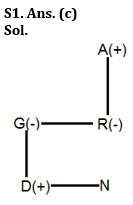S2. Ans. (e)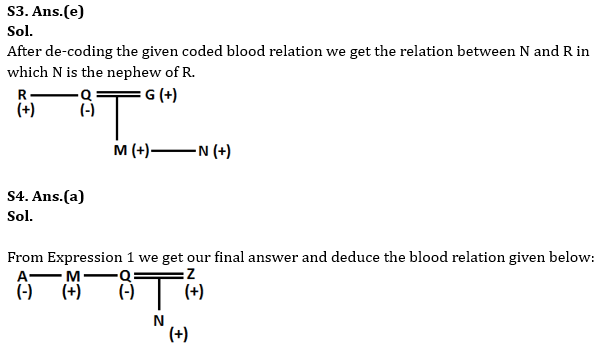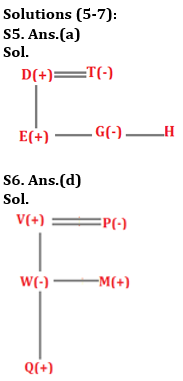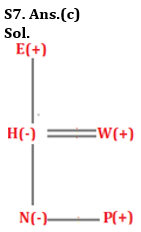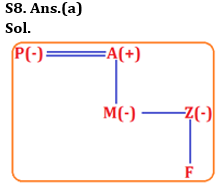S9. Ans.(e)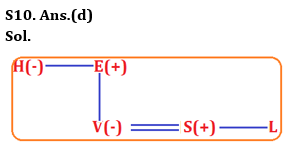## FAQs

### What is the selection process of the Bank Clerk?

There are 4 sections in the RBI Grade B Phase 1 Exam i.e. English Language, General Awareness, Quantitative Aptitude & Reasoning.

#### Congratulations!Union Budget 2023-24: Free PDF